Function Repository Resource:

# ComplexRootQ

Determine if a given number is an integer complex root of another number

Contributed by: Wolfram|Alpha Math Team

## Examples

### Basic Examples (4)

Test whether the given expression is a third root of unity:

 In:=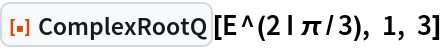Out=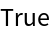Test whether the given expression is a sixth root of two:

 In:=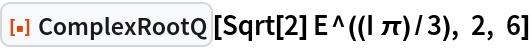Out=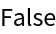Test whether a different expression is a sixth root of two:

 In:=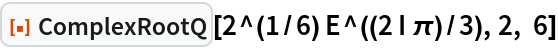Out=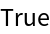Test whether the given expression is a sixth root of three:

 In:=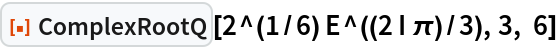Out=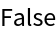### Properties and Relations (1)

Use ComplexRootQ to confirm the output of ResourceFunction["ComplexRoots"]:

 In:=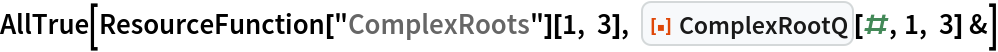Out=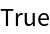## Publisher

Wolfram|Alpha Math Team

## Version History

• 4.0.0 – 23 March 2023
• 3.2.0 – 20 May 2021